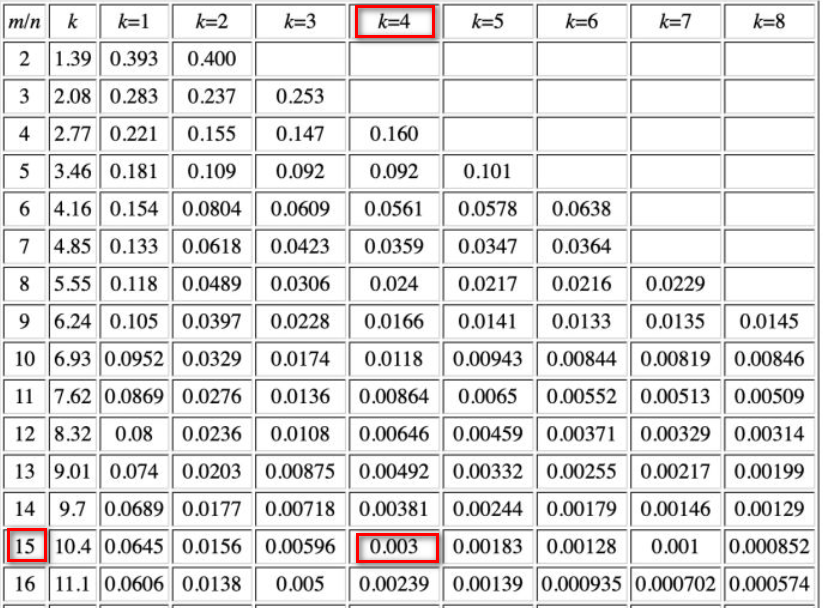# victoriaMetrics库之布隆过滤器

## victoriaMetrics库之布隆过滤器

### 概述

victoriaMetrics的`vmstorage`组件会接收上游传递过来的指标，在现实场景中，指标或瞬时指标的数量级可能会非常恐怖，如果不限制缓存的大小，有可能会由于cache miss而导致出现过高的slow insert

``````func getLabelsHash(labels []prompbmarshal.Label) uint64 {
bb := labelsHashBufPool.Get()
b := bb.B[:0]
for _, label := range labels {
b = append(b, label.Name...)
b = append(b, label.Value...)
}
h := xxhash.Sum64(b)
bb.B = b
labelsHashBufPool.Put(bb)
return h
}
``````

### 限速器的初始化

victoriaMetrics使用了一个类似限速器的概念，限制每小时/每天新增的唯一序列，但与普通的限速器不同的是，它需要在序列级别进行限制，即判断某个序列是否是新的唯一序列，如果是，则需要进一步判断一段时间内缓存中新的时序数目是否超过限制，而不是简单地在请求层面进行限制。

``````hourlySeriesLimiter = bloomfilter.NewLimiter(*maxHourlySeries, time.Hour)
dailySeriesLimiter = bloomfilter.NewLimiter(*maxDailySeries, 24*time.Hour)
``````

1. 初始化一个限速器，限速器的最大元素个数为`maxItems`
2. 则启用了一个goroutine，当时间达到`refreshInterval`时会重置限速器
``````func NewLimiter(maxItems int, refreshInterval time.Duration) *Limiter {
l := &Limiter{
maxItems: maxItems,
stopCh:   make(chan struct{}),
}
l.v.Store(newLimiter(maxItems)) //1
go func() {
defer l.wg.Done()
t := time.NewTicker(refreshInterval)
defer t.Stop()
for {
select {
case <-t.C:
l.v.Store(newLimiter(maxItems))//2
case <-l.stopCh:
return
}
}
}()
return l
}
``````

``````func (l *Limiter) Add(h uint64) bool {
}

func (l *limiter) Add(h uint64) bool {
if currentItems >= uint64(l.f.maxItems) {
return l.f.Has(h)
}
}
return true
}
``````

``````func newFilter(maxItems int) *filter {
bitsCount := maxItems * bitsPerItem
bits := make([]uint64, (bitsCount+63)/64)
return &filter{
maxItems: maxItems,
bits:     bits,
}
}
``````

``````	if p > 0.003 {
t.Fatalf("too big false hits share for maxItems=%d: %.5f, falseHits: %d", maxItems, p, falseHits)
}
``````1. 获取过滤器的完整存储，并转换为以bit单位
2. 将元素`h`转换为byte数组，便于xxhash.Sum64计算
3. 后续将执行hashesCount次哈希，降低漏失率
4. 计算元素h的哈希
5. 递增元素`h`，为下一次哈希做准备
6. 取余法获取元素的bit范围
7. 获取元素所在的slot(即uint64大小的bit范围)
8. 获取元素所在的slot中的bit位，该位为1表示该元素存在，为0表示该元素不存在
9. 获取元素所在bit位的掩码
10. 加载元素所在的slot的数值
11. 如果`w & mask`结果为0，说明该元素不存在，
12. 将元素所在的slot(`w`)中的元素所在的bit位(mask)置为1，表示添加了该元素
13. 由于`Add`函数可以并发访问，因此`bits[i]`有可能被其他操作修改，因此需要通过重新加载(14)并通过循环来在`bits[i]`中设置该元素的存在性
``````func (f *filter) Add(h uint64) bool {
bits := f.bits
maxBits := uint64(len(bits)) * 64 //1
bp := (*byte)(unsafe.Pointer(&h))//2
b := bp[:]
isNew := false
for i := 0; i < hashesCount; i++ {//3
hi := xxhash.Sum64(b)//4
h++ //5
idx := hi % maxBits //6
i := idx / 64 //7
j := idx % 64 //8
mask := uint64(1) << j //9
for (w & mask) == 0 {//11
wNew := w | mask //12
if atomic.CompareAndSwapUint64(&bits[i], w, wNew) {//13
isNew = true//14
break
}
}
}
return isNew
}
``````

``````func (f *filter) Has(h uint64) bool {
bits := f.bits
maxBits := uint64(len(bits)) * 64
bp := (*byte)(unsafe.Pointer(&h))
b := bp[:]
for i := 0; i < hashesCount; i++ {
hi := xxhash.Sum64(b)
h++
idx := hi % maxBits
i := idx / 64
j := idx % 64
mask := uint64(1) << j
if (w & mask) == 0 {
return false
}
}
return true
}
``````